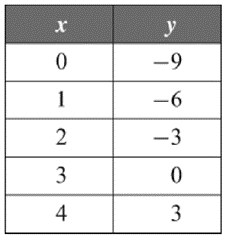Mathematics
Easy

Question

# Using the table of values, determine the equation of the line## y = –3x – 9y = –3x + 3y = 3x – 9y = 3x + 3Hint:

## The correct answer is: y = 3x – 9

### GIVEN-(0,-9), (1,-6), (2,-3), (3,0) and (4,3) are the solutions of a line.TO FIND-Equation of the line.SOLUTION-We take any 2 points from the given table, say- (0,-9) and (1,-6) to find the equation of the given line using 2 point formual.      (y-y1) = (y2-y1) * (x-x1)                    (x2-x1)∴ [y - (-9)] = [-6 - (-9)] * (x - 0)                      (1 - 0)∴    (y + 9) = (-6 + 9) * x                       1∴    (y + 9) = 3/1 * x∴       y + 9 = 3x∴             y = 3x - 9Final Answer:-Option 'c' i.e. 'y = 3x - 9' is the correct answer to the given question.

Alternatively, we can substitute all the values of x & y in the equations given and check whether LHS = RHS or not.
If all the points satisfy the given equation, that is the equation of the given line.
However, since the given table has 5 solutions for the equation, this method would be more time consuming. Hence, we follow the 2 point formula method.
However, final answer remains the same.

### Related Questions to study#### With Turito Foundation.#### Get an Expert Advice From Turito.